Drive_Index
Gears Gearboxes

Worm Gears

Introduction..... Nomenclature..... Design Parameters..... Specifications..... Designation..... Worm Gear Profiles..... Materials..... Backlash/quality..... Design Process.....
Thermal Design..... Worm Gear Formulue..... Friction Factors..... Efficiency..... Self Locking..... Strength Design to BS721..... Strength Design to AGMA.....

Introduction

A worm gear is used when a large speed reduction ratio is required between crossed axis shafts which do not intersect.   A basic helical gear can be used but the power which can be transmitted is low.  A worm drive consists of a large diameter worm wheel with a worm screw meshing with teeth on the periphery of the worm wheel.   The worm is similar to a screw and the worm wheel is similar to a section of a nut.   As the worm is rotated the wormwheel is caused to rotate due to the screw like action of the worm.  The size of the worm gearset is generally based on the centre distance between the worm and the wormwheel.

If the worm gears are machined basically as crossed helical gears the result is a highly stress point contact gear.  However normally the wormwheel is cut with a concave as opposed to a straight width.   This is called a single envelope worm gearset.   If the worm is machined with a concave profile to effectively wrap around the wormwheel the gearset is called a double enveloping worm gearset and has the highest power capacity for the size.  Single enveloping gearsets require accurate alignment of the worm-wheel to ensure full line tooth contact. Double enveloping gearsets require accurate alignment of both the worm and the wormwheel to obtain maximum face contact.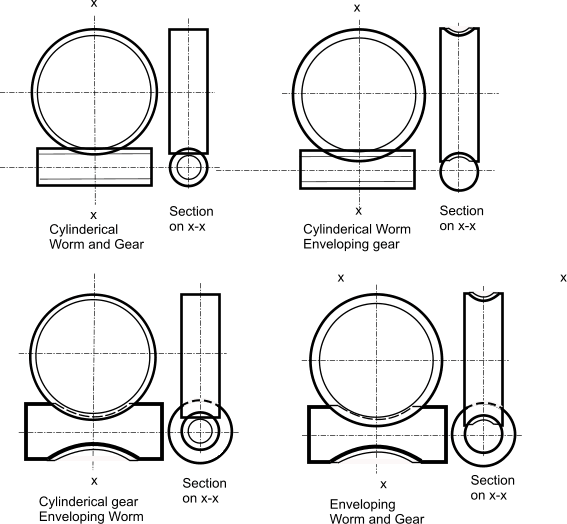Diagram showing the different worm gear options available.

The double enveloping (double throat/double globoid ) option is the most difficult to manufacture and set up.     However this option has the highest load capacity, near zero backlash capability, highest accuracy and extended life capability.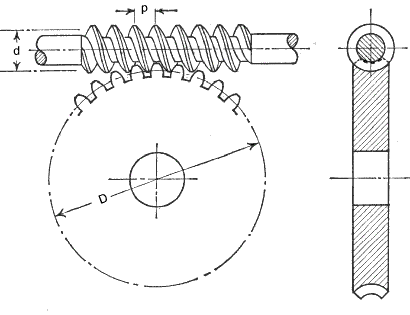A more detailed view showing a cylinderical worm and an enveloping gear.    The worm is shown with the worm above the wormwheel.  The gearset can also be arranged with the worm below the wormwheel.   Other alignments are used less frequently.

Nomenclature

As can be seen in the above view a section through the axis of the worm and the centre of the gear shows that , at this plane, the meshing teeth and thread section is similar to a spur gear and has the same features

αn = Normal pressure angle = 20o as standard
γ = Worm lead angle = (180 /π ) tan-1 (z 1 / q)(deg)   ..Note: for α n= 20o  γ should be less than 25o
b a = Effective face width of worm wheel. About 2.m (q +1) (mm)
b l = Length of worm wheel. About 14.m. (mm)
c = clearance   c min = 0,2.m cos γ ,  c max = 0,25.m cos γ (mm)
d 1 = Ref dia of worm (Pitch dia of worm (m)) = q.m (mm)
d a.1 = Tip diameter of worm = d 1 + 2.h a.1 (mm)
d 2 = Ref dia of worm wheel (Pitch dia of wormwheel) =( p x.z/π ) = 2.a - d 1 (mm)
d a.2 = Tip dia worm wheel (mm)
h f.1 = Worm Thread dedendum , min = m.(2,2 cos γ - 1 ) , max = m.(2,25 cos γ - 1 )(mm)
m = Axial module = p x /π (mm)
m n = Normal module = m cos γ(mm)
M 1 = Worm torque (Nm)
M 2 = Worm wheel torque (Nm)
n 1 = Rotational speed of worm (revs /min)
n 2 = Rotational speed of wormwheel (revs /min)
p x = Axial pitch of of worm threads and circular pitch of wheel teeth ..the pitch between adjacent threads = π. m. (mm)
p n = Normal pitch of of worm threads and gear teeth (m)
q = Worm diameter factor = d 1 / m - (Allows module to be applied to worm ) selected from (6   6,5   7   7,5   8   8,5   9  10   11   12   13   14   17   20 )
p z = Lead of worm = p x. z 1 (mm).. Distance the thread advances in one rev'n of the worm.   For a 2-start worm the lead = 2 . p x
R g = Reduction Ratio
μ = coefficient of friction
η= Efficiency
Vs = Worm-gear sliding velocity ( m/s)
z 1 = Number of threads (starts) on worm
z 2 = Number of teeth on wormwheel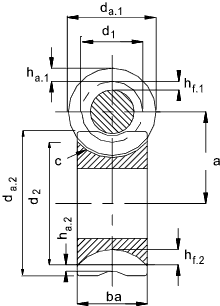Worm gear design parameters

Worm gears provide a normal single reduction range of 5:1 to 75-1.  The pitch line velocity is ideally up to 30 m/s.  The efficiency of a worm gear ranges from 98% for the lowest ratios to 20% for the highest ratios.  As the frictional heat generation is generally high the worm box must be designed disperse heat to the surroundings and lubrication is an essential requirement.  Worm gears are quiet in operation.  Worm gears at the higher ratios are inherently self locking - the worm can drive the gear but the gear cannot drive the worm.   A worm gear can provide a 50:1 speed reduction but not a 1:50 speed increase....(In practice a worm should not be used a braking device for safety linked systems e.g hoists.  . Some material and operating conditions can result in a wormgear backsliding )

The worm gear action is a sliding action which results in significant frictional losses.   The ideal combination of gear materials is for a case hardened alloy steel worm (ground finished) with a phosphor bronze gear.  Other combinations are used for gears with comparatively light loads.

Specifications

BS721 Pt2 1983 Specification for worm gearing � Metric units.
This standard is current (2004) and provides information on tooth form, dimensions of gearing, tolerances for four classes of gears according to function and accuracy, calculation of load capacity and information to be given on drawings.

Worm Gear Designation

Very simply a pair of worm gears can be defined by designation of the number of threads in the worm ,the number of teeth on the wormwheel, the diameter factor and the axial module i.e z1,z2, q, m .

This information together with the centre distance ( a ) is enough to enable calculation of and any dimension of a worm gear using the formulea available.

Worm teeth Profile

The sketch below shows the normal (not axial) worm tooth profile as indicated in BS 721-2 for unit axial module (m = 1mm) other module teeth are in proportion e.g. 2mm module teeth are 2 times larger

Typical axial modules values (m) used for worm gears are

0,5    0,6     0,8    1,0     1,25    1,6    2,0    2,5    3,15     4,0     5,0     6,3    8,0    10,0     12,5    16,0    20,0    25,0    32,0    40,0    50,0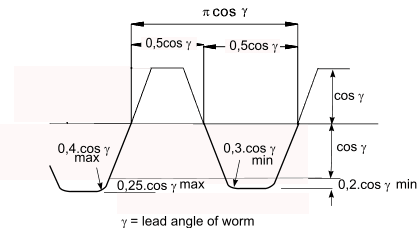Materials used for gears

 Material Notes applications Worm Acetal / Nylon Low Cost, low duty Toys, domestic appliances, instruments Cast Iron Excellent machinability, medium friction. Used infrequently in modern machinery Carbon Steel Low cost, reasonable strength Power gears with medium rating. Hardened Steel High strength, good durability Power gears with high rating for extended life Wormwheel Acetal /Nylon Low Cost, low duty Toys, domestic appliances, instruments Phos Bronze Reasonable strength, low friction and good compatibility with steel Normal material for worm gears with reasonable efficiency Cast Iron Excellent machinability, medium friction. Used infrequently in modern machinery

A worm gear set normally includes some backlash during normal manufacture to allow for expansion of the gear wheel when operating at elevated temperaturs.     The backlash is controlled by adusting the gear wheel tooth thickness.

BS 721 includes a table of backlash limits related to the accuracy grade.    The standard lists 5 accuracy grades.

AGMA and DIN provide a similar grading system

 Grade 1 relates to critical applications where minimum backlash is required i.e instruments /metering Grade 2 relates to precision drives such as machine tools Grade 3,4,5 relates to industrial drives with working temperatures of about 120o C

Design of a Worm Gear

The following notes relate to the principles in BS 721-2
Method associated with AGMA are shown below..

Initial sizing of worm gear.. (Mechanical)

1) Initial information generally Torque required (Nm), Input speed(rpm), Output speed (rpm).
2) Select Materials for worm and wormwheel.
3) Calculate Ratio (R g)
4) Estimate a = Center distance (mm)
5) Set z 1 = Nearest number to (7 + 2,4 SQRT (a) ) /R g
6) Set z 2 = Next number < R g . z 1
7) Using the value of estimated centre distance (a) and No of gear teeth ( z 2 )  obtain a value for q from the table below. (q -value selection)
8) d 1 = q.m (select) ..
9) d 2 = 2.a - d 1
10) Select a wormwheel face width b a (minimum =2*m*SQRT(q+1))
11) Calculate the permissible output torques for strength (M b_1 and wear M c_1 )
12) Apply the relevent duty factors to the allowable torque and the actual torque
13) Compare the actual values to the permissible values and repeat process if necessary
14) Determine the friction coefficient and calculate the efficiency.
15) Calculate the Power out and the power in and the input torque

16) Complete design of gearbox including design of shafts, lubrication, and casing ensuring sufficient heat transfer area to remove waste heat.

Initial sizing of worm gear.. (Thermal)

Worm gears are often limited not by the strength of the teeth but by the heat generated by the low efficiency. It is necessary therefore to determine the heat generated by the gears = (Input power - Output power). The worm gearbox must have lubricant to remove the heat from the teeth in contact and sufficient area on the external surfaces to distibute the generated heat to the local environment. This requires completing an approximate heat transfer calculation. If the heat lost to the environment is insufficient then the gears should be adjusted (more starts, larger gears) or the box geometry should be adjusted, or the worm shaft could include a fan to induced forced air flow heat loss.

Formulae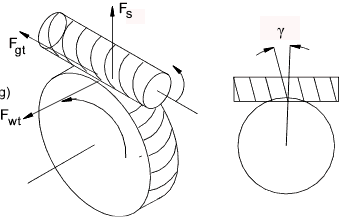The reduction ratio of a worm gear ( R g )

R g = z 2 / z 1

eg a 30 tooth wheel meshing with a 2 start worm has a reduction of 15

Tangential force on worm ( F wt )= axial force on wormwheel

F wt = F ga = 2.M 1 / d 1

Axial force on worm ( F wa ) = Tangential force on gear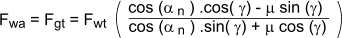Output torque ( M 2 ) = Tangential force on wormwheel * Wormwheel reference diameter /2

M 2 = F gt* d 2 / 2

Relationship between the Worm Tangential Force F wt and the Gear Tangential force F gt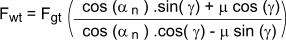Relationship between the output torque M 2and the input torque M 1

M 2 = ( M 1. d 2 / d 1 ).[ (cos α n - μ tan γ ) / (cos α n . tan (γ + μ) ) ]

Separating Force on worm-gearwheel ( F s )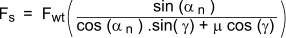Sliding velocity ( V s )...(m/s)

V s (m/s ) = 0,00005236. d 1. n 1 sec γ
= 0,00005235.m.n (z 12 + q 2 ) 1/2

Peripheral velocity of wormwheel ( V p) (m/s)

V p = 0,00005236,d 2. n 2

Friction Coefficient

Note: The values of the coeffient of friction as provided in the table below are based on the use of phosphor bronze wormwheels and case hardended , ground and polished steel worms , lubricated by a mineral oil having a viscosity of between 60cSt, and 130cSt at 60 deg.C .

Cast Iron and Phosphor Bronze .. Table x 1,15
Cast Iron and Cast Iron.. Table x 1,33
Quenched Steel and Aluminum Alloy..Table x 1,33
Steel and Steel..Table x 2

Friction coefficients - For Case Hardened Steel Worm / Phos Bros Wheel

 Sliding Speed Friction Coefficient Sliding Speed Friction Coefficient m/s μ m/s μ 0 0,145 1,5 0,038 0,001 0,12 2 0,033 0,01 0,11 5 0,023 0,05 0,09 8 0,02 0,1 0,08 10 0,018 0,2 0,07 15 0,017 0,5 0,055 20 0,016 1 0,044 30 0,016

Efficiency of Worm Gear

The efficiency of the worm gear is determined by dividing the output Torque M2 with friction = μ by the output torque with zero losses i.e μ = 0

First cancelling [( M 1. d 2 / d 1 ) / M 1. d 2 / d 1 ) ] = 1
Denominator = [(cos α n / (cos α n . tan γ ] = cot γ

η = [(cos α n - μ tan γ ) / (cos α n . tan γ + μ ) ] / cot γ

= [(cos α n - μ .tan γ ) / (cos α n + μ .cot γ )]

Graph showing worm gear efficiency related to gear lead angle ( γ )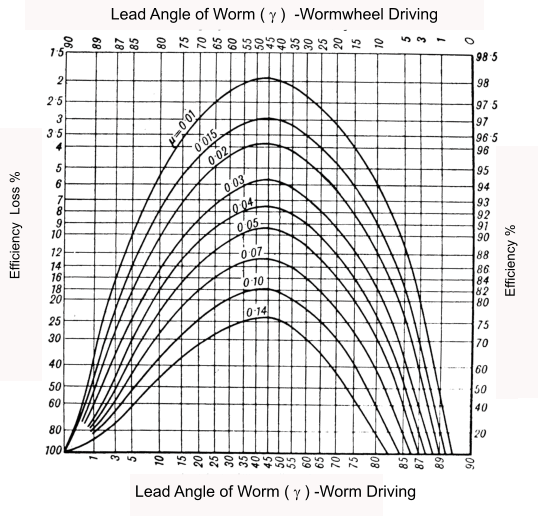Self Locking

Referring to the above graph , When the gear wheel is driving the curve points intersecting the zero efficiency line identify when the worm drive is self locking i.e the gear wheel cannot drive to worm.    It is the moment when gearing cannot be moved using even the highest possible torque acting on the worm gear.    The self-locking limit occurs when the worm lead angle ( γ ) equals atan (μ). (2o to 8o )

It is often considered that the static coefficient of friction is most relevant as the gear cannot be started.    However in practice it is safer to use the, lower, dynamic coefficient of friction as this comes into play if the gear set is subject to vibration.

Worm Design /Gear Wear / Strength Equations to BS721

Note: For designing worm gears to AGMA codes AGMA method of Designing Worm Gears

The information below relates to BS721 Pt2 1983 Specification for worm gearing � Metric units.  BS721 provides average design values reflecting the experience of specialist gear manufacturers.   The methods have been refined by addition of various application and duty factors as used.  Generally wear is the critical factor..

The permissible torque (M in Nm) on the gear teeth is obtained by use of the equation

M b = 0,0018 X b.2σ bm.2. m. l f.2. d 2.

( example 87,1 Nm = 0,0018 x 0,48 x 63 x 20 x 80 )

X b.2 = speed factor for bending (Worm wheel ).. See Below
σ bm.2 = Bending stress factor for Worm wheel.. See Table below
l f.2 = length of root of Worm Wheel tooth
d 2 = Reference diameter of worm wheel
m = axial module

Permissible Torque for Wear

The permissible torque (M in Nm) on the gear teeth is obtained by use of the equation

M c = 0,00191 X c.2σ cm.2.Z. d 21,8. m

( example 33,42 Nm = 0,00191 x 0,3234 x 6,7 x 1,5157 x 801,8 x 2 )

X c.2 = Speed factor for wear ( Worm wheel )
σ cm.2 = Surface stress factor for Worm wheel
Z = Zone factor.

Length of root of worm wheel tooth

Radius of the root = R r= d 1 /2 + h ha,1 (= m) + c(= 0,25.m.cos γ )
R r= d 1 /2 + m(1 +0,25 cosγ)

l f.2 = 2.R r.sin-1 (2.R r / b a)
Note: angle from sin-1(function) is in radians...

Speed Factor for Bending

This is a metric conversion from an imperial formula..
X b.2 = speed factor for bending = 0,521(V) -0,2

V= Pitch circle velocity =0,00005236*d 2.n 2 (m/s)

The table below is derived from a graph in BS 721. I cannot see how this works as a small worm has a smaller diameter compared to a large worm and a lower speed which is not reflected in using the RPM.

Table of speed factors for bending

 RPM (n2) X b.2 RPM (n2) X b.2 1 0,62 600 0,3 10 0,56 1000 0,27 20 0,52 2000 0,23 60 0,44 4000 0,18 100 0,42 6000 0,16 200 0,37 8000 0,14 400 0,33 10000 0,13

The formula for the acceptable torque for wear should be modified to allow additional factors which affect the Allowable torque M c

M c2 = M c. Z L. Z M.Z R / K C

The torque on the wormwheel as calculated using the duty requirements (M e) must be less than the acceptable torque M c2 for a duty of 27000 hours with uniform loading.   For loading other than this then M e should be modified as follows

M e2 = M e. K S* K H

Thus
uniform load < 27000 hours (10 years) M e M c2
Other conditions M e2 M c2

Factors used in equations

Lubrication (Z L)..
Z L = 1 if correct oil with anti-scoring additive else a lower value should be selected

Lubricant (Z M)..
Z L = 1 for Oil bath lubrication at V s < 10 m /s
Z L = 0,815 Oil bath lubrication at 10 m/s < V s < 14 m /s
Z L = 1 Forced circulation lubrication

Surface roughness (Z R ) ..
Z R = 1 if Worm Surface Texture < 3μ m and Wormwheel < 12 μ m
else use less than 1

Tooth contact factor (K C
This relates to the quality and rigidity of gears . Use 1 for first estimate
K C = 1 For grade A gears with > 40% height and > 50% width contact
= 1,3 - 1,4 For grade A gears with > 30% height and > 35% width contact
= 1,5-1,7 For grade A gears with > 20% height and > 20% width contact

Starting factor (K S) ..
K S =1 for < 2 Starts per hour
=1,07 for 2- 5 Starts per hour
=1,13 for 5-10 Starts per hour
=1,18 more than 10 Starts per hour

Time / Duty factor (K H) ..
K H for 27000 hours life (10 years) with uniform driver and driven loads
For other conditions see table below

Tables for use with BS 721 equations

Speed Factors

X c.2 = K V .K R
Note: This table is not based on the graph in BS 721-2 (figure 7) it is based on another more easy to follow graph.   At low values of sliding velocity and RPM it agrees closely with BS 721.   At higher speed velocities it gives a lower value (e.g at 20m/s -600 RPM the value from this table for X c.2 is about 80% of the value in BS 721-2

Table of Worm Gear Speed Factors

Note -sliding speed = Vs and Rotating speed = n2 (Wormwheel)

 Sliding speed K V Rotating Speed K R m/s rpm 0 1 0,5 0,98 0,1 0,75 1 0,96 0,2 0,68 2 0,92 0,5 0,6 10 0,8 1 0,55 20 0,73 2 0,5 50 0,63 5 0,42 100 0,55 10 0,34 200 0,46 20 0,24 500 0,35 30 0,16 600 0,33

Stress Factors

Table of Worm Gear Stress Factors
 Other metal(Worm) P.B. C.I. 0,4%C.Steel 0,55%C.Steel C.Steel   Case. H'd Metal(Wormwheel) Bending(σbm ) Wear ( σ cm ) MPa MPa Phosphor BronzeCentrifugal cast 69 8,3 8,3 9,0 15,2 Phosphor BronzeSand Cast Chilled 63 6,2 6,2 6,9 12,4 Phosphor BronzeSand Cast 49 4,6 4,6 5,3 10,3 Grey Cast Iron 40 6,2 4,1 4,1 4,1 5,2 0,4% Carbon steel 138 10,7 6,9 0,55% Carbon steel 173 15,2 8,3 Carbon Steel (Case hardened) 276 48,3 30,3 15,2

Zone Factor (Z)

If b a < 2,3 (q +1)1/2 Then Z = (Basic Zone factor ) . b a /2 (q +1)1/2
If b a > 2,3 (q +1)1/2 Then Z = (Basic Zone factor ) .1,15

Table of Basic Zone Factors
 q z1 6 6,5 7 7,5 8 8,5 9 9,5 10 11 12 13 14 17 20 1 1,045 1,048 1,052 1,065 1,084 1,107 1,128 1,137 1,143 1,16 1,202 1,26 1,318 1,402 1,508 2 0,991 1,028 1,055 1,099 1,144 1,183 1,214 1,223 1,231 1,25 1,28 1,32 1,36 1,447 1,575 3 0,822 0,89 0,989 1,109 1,209 1,26 1,305 1,333 1,35 1,365 1,393 1,422 1,442 1,532 1,674 4 0,826 0,83 0,981 1,098 1,204 1,701 1,38 1,428 1,46 1,49 1,515 1,545 1,57 1,666 1,798 5 0,947 0,991 1,05 1,122 1,216 1,315 1,417 1,49 1,55 1,61 1,632* 1,652 1,675 1,765 1,886 6 1,131 1,145 1,172 1,22 1,287 1,35 1,438 1,521 1,588 1,625 1,694 1,714 1,733 1,818 1,928 7 1,316 1,34 1,37 1,405 1,452 1,54 1,614 1,704 1,725 1,74 1,76 1,846 1,98 8 1,437 1,462 1,5 1,557 1,623 1,715 1,738 1,753 1,778 1,868 1,96 9 1573 1,604 1,648 1,72 1,743 1,767 1,79 1,88 1,97 10 1,68 1,728 1,748 1,773 1,798 1,888 1,98 11 1,732 1,753 1,777 1,802 1,892 1,987 12 1,76 1,78 1,806 1,895 1,992 13 1,784 1,806 1,898 1,998 14 1,811 1,9 2

Duty Factor

Duty - time Factor K H
 Impact from Prime mover Expected life hours K H Impact From Load Uniform Load Medium Impact Strong impact Uniform Load Motor Turbine Hydraulic motor 1500 0,8 0,9 1 5000 0,9 1 1,25 27000 1 1,25 1,5 60000 1,25 1,5 1,75 Light impactmulti-cylinder engine 1500 0,9 1 1,25 5000 1 1,25 1,5 27000 1,25 1,5 1,75 60000 1,5 1,75 2 Medium Impact Single cylinder engine 1500 1 1,25 1,5 5000 1,25 1,5 1,75 27000 1,5 1,75 2 60000 1,75 2 2,25

Worm q value selection

The table below allows selection of q value which provides a reasonably efficient worm design.   The recommended centre distance value "a" (mm)is listed for each q value against a range of z 2 (teeth number values).   The table has been produced by reference to the relevant plot in BS 721
Example
If the number of teeth on the gear is selected as 45 and the centre distance is 300 mm then a q value for the worm would be about 7.5

Important note: This table provides reasonable values for all worm speeds. However at worm speeds below 300 rpm a separate plot is provided in BS721 which produces more accurate q values.    At these lower speeds the resulting q values are approximately 1.5 higher than the values from this table. The above example at less than 300rpm should be increased to about 9

Table of Center distances "a" relating to q values and Number of teeth on Worm gear z 2

 Number of Teeth On Worm Gear (z 2) q 20 25 30 35 40 45 50 55 60 65 70 75 80 6 150 250 380 520 700 6.5 100 150 250 350 480 660 7 70 110 170 250 350 470 620 700 7.5 50 80 120 180 240 330 420 550 670 8 25 50 80 120 180 230 300 380 470 570 700 8.5 28 90 130 130 180 220 280 350 420 500 600 700 9 40 70 100 130 170 220 280 330 400 450 520 9.5 25 50 70 100 120 150 200 230 300 350 400 10 26 55 80 100 130 160 200 230 270 320 11 25 28 55 75 100 130 150 180 220 250 12 28 45 52 80 100 130 150 100 13 27 45 52 75 90 105

AGMA method of Designing Worm Gears

The AGMA method is provided here because it is relatively easy to use and convenient- AGMA is all imperial and so I have used conversion values so all calculations can be completed in metric units..

Good proportions indicate that for a centre to centre distance = C the mean worm dia d 1 is within the range
Imperial (inches)

( C 0,875 / 3 )     d 1      ( C 0,875 / 1,6 )

Metric ( mm)

( C 0,875 / 2 )     d 1      ( C 0,875 / 1,07 )

The acceptable tangential load (W t) all

(W t) all = C s. d 20,8 .b a .C m .C v . (0,0132) (N)

The formula will result in a life of over 25000 hours with a case hardened alloy steel worm and a phosphor bronze wheel

C s = Materials factor
b a = Effective face width of gearwheel = actual face width. but not to exceed 0,67 . d 1
C m = Ratio factor
C v = Velocity factor

Modified Lewis equation for stress induced in worm gear teeth .

σ a = W t / ( p n. b a. y )(N)

W t = Worm gear tangential Force (N)
y = 0,125 for a normal pressure angle α n = 20o

The friction force = W f

W f = f.W t / (. cos φ n ) (N)

γ = worm lead angle at mean diameter
α n = normal pressure angle

The sliding velocity = V s

V s = π .n 1. d 1 / (60,000 )

d 1 = mean dia of worm (mm)
n 1 = rotational speed of worm (revs/min)

The torque generated γ at the worm gear = M b (Nm)

T G = W t .d 1 / 2000

The required friction heat loss from the worm gearbox

H loss = P in ( 1 - η )

η = gear efficiency as above.

C s values

C s = 270 + 0,0063(C )3... for C 76mm ....Else

C s (Sand cast gears ) = 1000 for d 1 64 mm ...else... 1860 - 477 log (d 1 )

C s (Chilled cast gears ) = 1000 for d 1 200 mm ...else ... 2052 -456 log (d 1 )

C s (Centrifugally cast gears ) = 1000 for d 1 635 mm ...else ... 1503 - 180 log (d 1 )

C m values

NG = Number of teeth on worm gear.
NW = Number of starts on worm gear.
mG = gear ration = NG /NW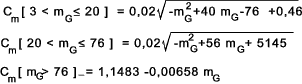C v values

C v (V s > 3,56 m/s ) = 0,659 exp (-0,2167 V s )

C v (3,56 m/s V s < 15,24 m/s ) = 0,652 (V s) -0,571 )

C v (V s > 15,24 m/s ) = 1,098.( V s ) -0,774 )

f values

f (V s = 0) = 0,15

f (0 < V s 0,06 m/s ) = 0,124 exp (-2,234 ( V s ) 0,645

f (V s > 0,06 m/s ) = 0,103 exp (-1,1855 ( V s ) ) 0,450 ) +0,012

 Links to Gear Design Reynold ...A highly regarded gear manufacturer SEW Eurodrive...All the information on Gearboxes you will need Quality Transmission Components...Supplier with downloadable Gear Design Handbook Stock Drive Products= Sterling Instruments...Supplier with large quantity of downloadable drive information Lenze...Drive system supplier with geared motor section Mitcalc...Excel based software including coded gear design

Drive_Index
Gears Gearboxes